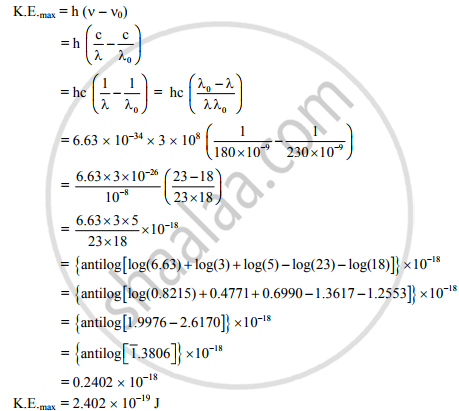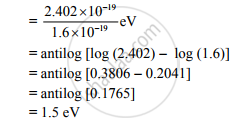# Determine the Maximum Kinetic Energy in Joule and in Ev of the Ejects Electron for the Metal Surface When It is Exposed to a Radiation of Wavelength 180 Nm. - Physics

The photoelectric threshold wavelength of a metal is 230 nm. Determine the maximum kinetic energy in joule and in eV of the ejects electron for the metal surface when it is exposed to a radiation of wavelength 180 nm.

[Planck’s constant : h = 6.63 * 10-34 Js, Velocity of light : C = 3 * 108 m/s.]

#### Solution

λ0 = 230 nm = 230 × 10−9m,

λ = 180 nm = 180 × 10−9m,
h = 6.63 × 10−34 J s, c = 3 × 108
m/s, K.E. (in joule and eV) = ?
Now,∴ The maximum kinetic energy of the ejected electron is 2.402 × 10−19 J.∴ The maximum kinetic energy of the ejected electron is 1.5 eV.

Concept: Einstein’s Equation - Particle Nature of Light
Is there an error in this question or solution?
2013-2014 (October)

Share